PHY_10_40_V1_DM_Internal r
Оценка 4.7

# PHY_10_40_V1_DM_Internal r

Оценка 4.7
docx
07.05.2020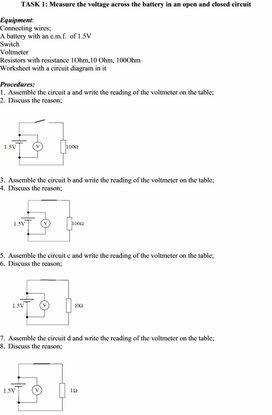PHY_10_40_V1_DM_Internal r.docx

TASK 1: Measure the voltage across the battery in an open and closed circuit

Equipment:

Connecting wires;

A battery with an e.m.f.  of 1.5V

Switch

Voltmeter

Resistors with resistance 1Ohm,10 Ohm, 100Ohm

Worksheet with a circuit diagram in it

Procedures:

1.    Assemble the circuit a and write the reading of the voltmeter on the table;

2.    Discuss the reason;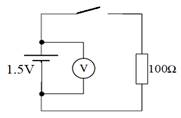3.    Assemble the circuit b and write the reading of the voltmeter on the table;

4.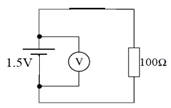Discuss the reason;

5.    Assemble the circuit c and write the reading of the voltmeter on the table;

6.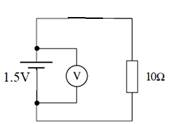Discuss the reason;

7.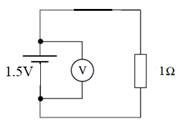Assemble the circuit d and write the reading of the voltmeter on the table;

8.    Discuss the reason;

Result table:

 Circuit a Circuit b Circuit c Circuit d V= V= V= V= R=100Ohm R=100Ohm R=10Ohm R=1Ohm I observed ________     The reason is ____

Скачать файл